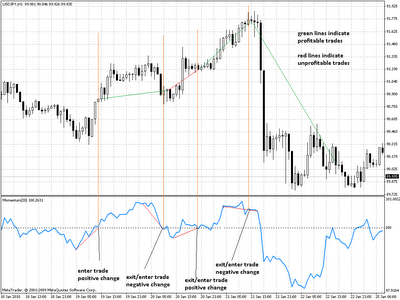## The Indicator Series : The Momentum Indicator

On the last post I wrote on the indicator series we discussed the MACD indicator and how it can be thought of as the “market’s speedometer”. Today we are going to talk about another very interesting indicator which tries to show us the prevalent direction of market change an instrument is going through. Within the next few paragraphs I will talk about the momentum indicator, its mathematical basis and some possible ways in which it could be implemented successfuly in an automated trading strategy.

The momentum indicator is a very simple technical tool which tries to show us a comparison between the price of the current bar (open, close, high or low can be used) and the same price of a given number of periods in the past. The indicator is calculated according to the following formula :

Momentum = (Price of Current Bar / Price N periods)*100

As you can see, what the indicator does is merely a calculation of the fraction represented by current price of the price of N precious periods. By multiplying this fraction by 100 the indicator effectively represents a percetange of current price against previous price levels. This means that if we calculate the 14 period momentum indicator on close price and the value of momentum is 102 it means that the closing price of the current bar is 2% higher than the average closing price of the last 14 periods.

As you may see by now, the usefulness of the momentum indicator by itself is not pretty straightforward as you are only using information comparing the current bar to a given N number of periods in the past and you may get a lot of false signals just because price on the current bar is higher than average. The traditional way to trade the momentum indicator is to get into positions as the indicator crosses the 100% line which effectively indicates that current price is now higher than previous price levels, however this often leads to late entries since the momentum indicator gives a signal after significant movements have happened and therefore this approach is only profitable when price action is particularly slow.

There are several ways to deal with this problem to create a more profitable system based on the momentum indicator. Particuarly we could base decisions on a derivative of the momentum indicator and enter when momentum starts to become positive. This will effectively get us into trades when the close of bars are becoming higher ignoring the fact that they may be above or below the average of the previous N periods. However the fact that the price of the current bar has heavy importance in the calculation of the indicator makes it a noisy one and the first derivative would have to be smoothed so that it didn’t use differences between current and last bar values of momentum but the difference between the current and some N past bar. The image below shows a few trades using this concept.If we follow this concept then it may become simpler to actually calculate the second derivative of momentum and simply enter or exit trades when the second derivative of momentum crosses the 0 line, effectively signaling that a change in market direction is starting to occur far before we actually see current price levels above or below the 0 line.